# Trigonometric Function

Also found in: Dictionary, Thesaurus, Wikipedia.
Related to Trigonometric Function: exponential function, Inverse trigonometric function

## Trigonometric Function

Trigonometric functions make up one of the most important classes of elementary functions.Figure 1

To define the trigonometric functions, we may consider a circle of unit radius with two mutually perpedicular diameters A’A and B’B (Figure 1). Arcs of arbitrary length are plotted from point A along the perimeter. If the arcs are laid out in the direction from A to B, that is, counterclockwise, they are regarded as positive; if they are laid out in the direction from A to B’, that is, clockwise, they are considered negative. If C is the end point of an arc of length ɸ, then the projection OP of the radius OC on the diameter A’A is called the cosine of the arc ɸ, and we write OP = cos ɸ. Here, by the projection OP we mean the length of the directed line segment OP; the length has a plus sign if P lies on O A and a minus sign if P lies on OA’. The projection OQ of the radius OC on the diameter B’B is called the sine of the arc i>, and we write OQ = sin ɸ. Here, a plus sign is used if Q lies on OB, and a minus sign if Q lies on OB’. The absolute values of the trigonometric functions cos ɸ and sin ɸ cannot be greater than 1; that is,

ǀcos ɸǀ ≤ 1 ǀsin ɸǀ ≤ 1

Cos ɸ and sin ɸ can also be defined as the rectangular Cartesian coordinates of the point C on a circle of unit radius whose center is located at the origin of coordinates. In this case, the diameter A’A lies on the axis of abscissas, and the diameter B’B lies on the axis of ordinates.

Since the radius of the circle is unity, the central angle in radian measurement is measured by the same number as the arc. Consequently, cos ɸ and sin ɸ may be considered the cosine and sine of an angle. In general, the argument of a trigonometric function is considered to be a number, which may be regarded geometrically as the length of an arc or the radian measure of an angle. If the argument is regarded as an angle, the argument’s value can also be expressed in degree measure. For acute angles ɸ (0 < ɸ < π/2), and only for such angles, the functions cos ɸ and sin ɸ may be defined with reference to a right triangle one of whose angles is ɸ. Thus, cos ɸ is the ratio of the leg adjacent to ɸ and the hypotenuse, and sin ɸ is the ratio of the leg opposite ɸ and the hypotenuse.

The arc AB of the circle is called the first quadrant of the circle, and the arcs BA’, A’B’, and B’A are called the second, third, and fourth quadrants, respectively. For angles ɸ in the first quadrant, cos ɸ > 0 and sin ɸ > 0; for angles in the second quadrant, cos ɸ < 0 and sin ɸ > 0; for angles in the third quadrant, cos ɸ < 0 and sin ɸ < 0; and for angles in the fourth quadrant, cos ɸ > 0 and sin ɸ < 0. Furthermore, cos ɸ is an even function—that is, cos (– ɸ) = cos ɸ. On the other hand, sin ɸ is an odd function: sin (–ɸ) = –sin ɸ.

Other trigonometric functions can be defined in terms of the basic trigonometric functions sin ɸ and cos ɸ. The most important such functions are the tangent (tan), cotangent (cot, or ctn), secant (sec), and cosecant (csc):The functions tan ɸ and sec ɸ are defined only for those ɸ for which cos ɸ ≠ 0, and cot ɸ and csc ɸ are defined only for those ɸ for which sin ɸ ≠ 0. The function sec ɸ is even, and the functions csc ɸ, tan ɸ, and cot ɸ are odd. Like cos ɸ and sin ɸ, these functions can be represented geometrically by line segments. In Figure 1, for example, tan ɸ = AL, cot ɸ = BK, sec ɸ = OL, and csc ɸ = OK. (Here, it is assumed that ɸ is acute; the trigonometric functions of other angles can be represented by the corresponding line segments for such angles.)

The names of the trigonometric functions derive from the functions’ geometric representations. For example, the term “tangent” comes from the Latin tangens, which means touching; in Figure 1 tan ɸ is represented by the segment AL of a line that touches, or is tangent to, the circle. The term “secant” comes from the Latin secans, which means cutting; sec ɸ is represented by the segment OL of a line that cuts the circle. The term “sine” derives from the Latin sinus, which means fold or bosom and which was used as a translation of the Arabic jaib. The Hindu mathematicians had used the term jiva, which means bowstring, to refer to the sine. The Arabs rendered ¡iva as ¡iba and subsequently used the reading jaib, which is a common word in Arabic. The terms “cosine, ” “cotangent, ” and “cosecant” derive from shortened forms of the term complementi sinus (sine of the complement) and similar terms: for angles ɸ up to π/2 (or, in degree measure, 90°) cos ɸ, cot ɸ, and csc ɸ are equal to the sine, tangent, and secant, respectively, of the complement of ɸ. Thus, we have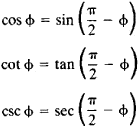Like the sine and cosine, the other trigonometric functions of acute angles may be regarded as the ratios of the lengths of the sides of a right triangle. The tangent is the ratio of the opposite leg and the adjacent leg, the cotangent is the ratio of the adjacent leg and the opposite leg, the secant is the ratio of the hypotenuse and the adjacent leg, and the cosecant is the ratio of the hypotenuse and the opposite leg.

The end point C of the arc ɸ is also the end point of the arcs ɸ + 2π, ɸ + 4π, . . ., since the circumference is 2π. Consequently, all trigonometric functions are periodic. The fundamental period of the functions sin ɸ, cos ɸ, sec ɸ, and csc ɸ is the number 2π, which corresponds to an angle of 360°; the fundamental period of tan ɸ and cot ɸ is the number π, which represents an angle of 180°. The graphs of these trigonometric functions are illustrated in Figure 2.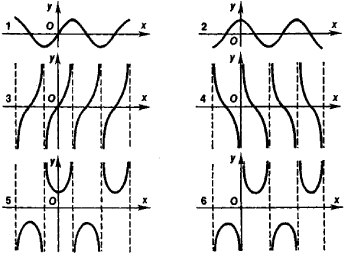Figure 2. Graphs of trigonometric functions: (1) sine, (2) cosine, (3) tangent, (4) cotangent, (5) secant, (6) cosecant

The following relations, sometimes called the Pythagorean . identities, hold for trigonometric functions having the same argument:

sin2 ɸ + cos2 ɸ = 1

tan2 ɸ + 1 = sec2 ɸ

cot2 ɸ + 1 = csc2 ɸ

For some values of the argument, the values of the trigonometric functions can be obtained from geometric considerations (see Table 1).

For large values of the argument, identities called reduction formulas may be used. The reduction formulas make it possible to express the trigonometric functions of any argument in terms of the trigonometric functions of an argument ɸ such that 0 ≤ ɸ ≤ π/2 or even 0 ≤ ɸ ≤ π/4. This circumstance simplifies the construction and use of tables of trigonometric functions and the

Table 1. Values of trigonometric functions for certain values of the argument ɸ
ɸɸTrigonometric functions
00010nonexistent1nonexistent
30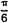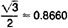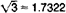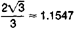2
45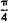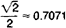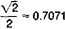11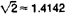60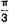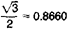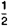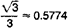290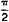10nonexistent0nonexistent1

construction of graphs. The reduction formulas have the form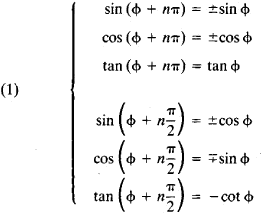In the first three formulas n may be any integer; the upper sign is used when n = 2k, and the lower sign when n = 2k + 1. In the second three formulas n may be only an odd number; the upper sign is used when n = 4k + 1, and the lower sign when n = 4k – 1.

Another important group of identities consists of addition formulas, which express the trigonometric functions of the sum or difference of the values of the argument in terms of the trigonometric functions of these values:In each formula either the upper signs or the lower signs are to be used throughout; that is, the upper sign on the right side of the formula corresponds to the upper sign on the left side, and the lower sign on the right side corresponds to the lower sign on the left side.

Formulas for the trigonometric functions of multiple arguments can be derived from the addition formulas—for example,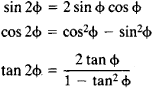The above identities are often called double-angle formulas. Formulas that express the powers of the sine and cosine of an argument in terms of the sine and cosine of multiples of the argument are frequently useful. Examples are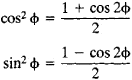The formulas for cos2 ɸ and sin2 ɸ may be used to find the values of the trigonometric functions of a half argument: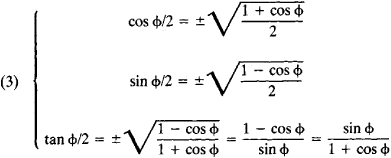Equations (3) are called half-angle formulas. The sign before the root symbol is selected according to the value of ɸ/2.

The sums or differences of trigonometric functions of different arguments can be converted into products according to the following formulas: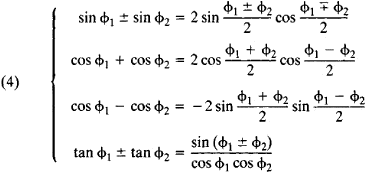Here, in the first formula and in the last formula either the upper signs or the lower signs are used throughout.

The products of trigonometric functions can be converted into a sum or difference by means of the formulas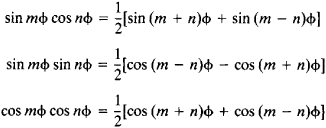The derivatives of the trigonometric functions can be expressed in terms of trigonometric functions:The integrals of the trigonometric functions are trigonometric functions or logarithms of trigonometric functions: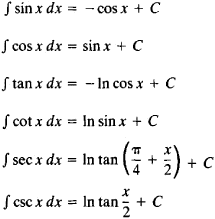The integrals of rational combinations of trigonometric functions are always elementary functions.

All trigonometric functions can be expanded in power series. The functions sin x and cos x can be expressed by series that converge for all values of x: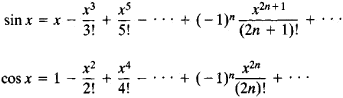These series can be used to obtain approximate expressions for sin x and cos x for small values of x:The trigonometric system 1, cos x, sin x, cos 2x, sin 2x, . . ., cos nx, sin nx, . . . constitutes an orthogonal system of functions on the interval [– π, π]. This fact makes it possible to represent functions by trigonometric series.

For complex values of the argument, the values of trigonometric functions can be determined by means of power series. The relation between trigonometric functions of a complex argument and the exponential function is given by Euler’s formula:

eiz = cos z + i sin z

From this formula expressions can be obtained for sin x and cos x in terms of the exponential functions of a purely imaginary argument: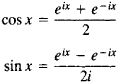These equations, which also are called Euler’s formulas, can be used to determine the values of cos z and sin z for complex z. For purely imaginary values z = ix (where x is real), we obtainwhere cosh x and sinh x are the hyperbolic cosine and hyperbolic sine, respectively (seeHYPERBOLIC FUNCTIONS). Moreover,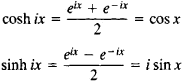The sine and cosine of a complex argument may assume real values that exceed 1 in absolute value. For example,The trigonometric functions of a complex argument are analytic functions. Furthermore, sin z and cos z are entire functions, and tan z, cot z, sec z, and csc z are meromorphic functions. The poles of tan z and sec z are at the points, and the poles of cot z and cscz are at the points z = πn; here, n = 0, ±1, ±2,. . . . The analytic function w = sin z maps the half strip – π < x < π, y > 0 of the z-plane conformally onto the w-plane outside the interval on the real axis between the points – 1 and +1. Moreover, the families of rays x = x0 and intervals y = y0 are mapped into families of confocal hyperbolas and ellipses, respectively. The strip, which is half as wide, is mapped into the upper half plane.

The equation x = sin y defines y as a multiple-valued function of x. This function is the inverse of the sine and is symbolized Arc sin x. The inverse functions of the cosine, tangent, cotangent, secant, and cosecant are defined in a similar way; they are Arc cos x, Arc tan x, Arc cot x, Arc sec x, and Arc csc x. Such functions are called inverse trigonometric functions. In non-Russian mathematical literature they are sometimes denoted sin–1 z, cos–1 z, and so on.

Trigonometric functions were first used in connection with studies in astronomy and geometry. The relations among line segments in a triangle and circle are in essence trigonometric functions, and these relations were employed as early as the third century B.C. by such ancient Greek mathematicians as Euclid, Archimedes, and Apollonius of Perga. The relations, however, did not constitute an independent subject of study for these mathematicians; consequently, it cannot be said that trigonometric functions as such were investigated by them. Trigonometric functions were originally treated as line segments and were used in this form in the solution of spherical triangles by Aristarchus (late fourth and second half of third centuries B.C.), Hipparchus (second century B.C.), Menelaus (first century A.D.), and Ptolemy (second century A.D.).

Ptolemy constructed the first table of chords for acute angles; it gave the chords at intervals of 30’ to an accuracy of 10~6 and was in effect the first table of sines. Aryabhata (late fifth century) was apparently the first to treat the function sin ɸ as a ratio. The first instances of tan ɸ and cot ɸ are provided by al-Battani (second half of ninth and early tenth centuries) and Abu al-Wafa (tenth century); Abu al-Wafa also used sec ɸ and csc ɸ. Aryabhata knew the formula sin2 ɸ + cos2 ɸ = 1, as well as formulas (3), which he used to construct a table of sines at intervals of 3°45’ on the basis of the known values of the trigonometric functions for simple arguments (π/3, π/6). Bhaskara (12th century) gave a method of constructing tables at intervals of 1° through the use of formulas (2). Formulas (4) were derived by Regiomontanus (15th century) and by J. Napier in connection with his invention of logarithms (1614). Regiomontanus produced a table of sines at intervals of 1’. I. Newton obtained expansions of trigonometric functions in power series (1669).

The theory of trigonometric functions was given its modern form by L. Euler (18th century). He defined trigonometric functions for real and complex arguments, provided the notation now used, established the relation between the exponential function and trigonometric functions, and showed the orthogonality of the system of sines and cosines.

### REFERENCES

Kochetkov, E. S., and E. S. Kochetkova. Algebra i elementarnye funktsii, parts 1–2. Moscow, 1966.
Shabat, B. V. Vvedenie v kompleksnyi onaliz. Moscow, 1969. Pages 61–65.
References in periodicals archive ?
Delay from source S (phased array element) to target T (defect) was calculated using conventional methods and trigonometric functions with respect to angles or by Fermat principle to express as the shortest path problem .
When we detect the seismic wave with a laser interferometer, the seismic signal can be expressed in terms of the intensity of the laser signal in a trigonometric function. The equation can be represented as follows:
 applied the new improved Riccati equation method to system (44) and then found that its exact solutions were expressed in terms of fractions of trigonometric functions and fractions of hyperbolic functions.
Waseem, "Shape-preserving trigonometric functions," Computational and Applied Mathematics, 2013.
Kantabutra, On hardware for computing exponential and trigonometric functions, IEEE Transactions on Computers, Vol.
The effect of the parameters in a trigonometric function on the size of the period and other properties is clearly seen in movie 2 (see Figure 2) and can be manipulated by entering the interactive work sheet.
A very interesting property of these is that there are exponential generating functions linked with trigonometric functions. The theorem below is also a wide generalization of the result in (2), which is related with a q-analog of secant numbers having exponential generating function sec(z).
Note, however, that the obtained optimum results for NTPs with functions A, B and F in Table 18 and Table 19 are presented only for global solvers LINDOGlobal and LGO since BARON could not handle trigonometric functions within the optimization model.
(2.8), (3.3), (3.5), (3.8) and (3.9) we obtain the trigonometric function solution of Eq.
The west, too, had equally dedicated zealots; Rheticus, who was mentored by Copernicus, along with a team of four others, in a labor of twelve years, generated 388,800 entries of tables for the six standard trigonometric functions to fifteen significant digits in the last seven hundred pages of his Opus Palatinum.
For some students numbers fall gently and expertly into equations with little gnashing of the teeth; for others phrases like irrational numbers one-to-one function and inverse trigonometric function are enough to cause spasms of confusion self-doubt and mental paralysis.
One exception was Georg Simon Klugel (1739-1812) who introduced a relatively modern concept of trigonometric function in  1770.

Site: Follow: Share:
Open / Close